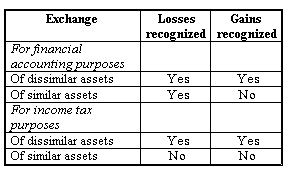Subject 6. Derecognition
#cfa #cfa-level-1 #financial-reporting-and-analysis #has-images #inventories-long-lived-assets-income-taxes-and-non-current-liabilities #long-lived-assets
For accounting purposes, an asset may be disposed of in three different ways. It may be:

• sold for cash
• exchanged for another asset
• abandoned

When plant assets are disposed of, depreciation should be recorded on the date of disposal. The cost is then removed from the asset account and the total recorded depreciation is removed from the accumulated depreciation account. Normally an asset's market value at the time of sale or disposal will most likely be different than the asset's book value (its original historical cost minus all accumulated depreciation on that asset). The sale of a plant asset at a price above or below book value results in a gain or loss to be reported in the income statement.

Because different depreciation methods are used for income tax purposes, the gain or loss reported on income tax returns may differ from that shown in the income statement. It is the gain or loss shown in the financial statement that is recorded in the company's general ledger accounts.

To illustrate each of these methods consider this. A machine was purchased on 1 January Year 1 for $1,000. The depreciation method was straight-line with a useful-life of 5 years and an estimated residual value of$200. On 31 July Year 3 the firm decides to dispose of the asset. The firm has a December year-end.

The first step irrespective of the method of disposal is to calculate the depreciation up to the date of sale:

Depreciation per year = (Cost - residual value) / Useful life = (1,000 - 200) / 5 = $160 Depreciation for year 1 =$160
Depreciation for year 2 = $160 Depreciation for year 3 =$93 (160 x 7/12)
Total: $413 Remember that the depreciation for year 3 is only for 7 months, as the asset is disposed of on 31 July Year 3. Sale of Long-Lived Assets The gain or loss on the sale of long-lived assets is computed as the sales proceeds minus the carrying value of the asset at the time of sale. Assume that the machinery is sold for cash in three scenarios: a. Sold for$587 cash (Sale of machinery for carrying value)
Debit: Cash (B/S) $587 Debit: Accumulated depreciation (B/S)$413
Credit: Machinery (B/S) $1000 b. Sold for$600 cash (Sale of machinery for above carrying value)
Debit: Cash (B/S) $600 Debit: Accumulated depreciation (B/S)$413
Credit: Machinery (B/S) $1000 Credit: Profit on sale of machinery (I/S)$13

c. Sold for $500 cash (Sale of machinery for below carrying value) Debit: Cash (B/S)$500
Debit: Accumulated depreciation (B/S) $413 Debit: Loss on sale of machinery (I/S)$87
Credit: Machinery (B/S) $1000 In summary, when disposing of an asset, entries are prepared to: • eliminate the cost of the asset from the books. • eliminate the accumulated depreciation from the books. • record the proceeds on the sale. This is reported as cash from investing activities on the statement of cash flows. • record the profit or loss on the sale (if applicable). This amount is excluded from net income when the indirect method is used to calculate cash flows from operating activities. Exchange of Long-Lived Assets If an asset is exchanged for another asset, the basic accounting is similar to the accounting for sales of plant assets for cash. If the trade-in allowance received is greater than the carrying value of the asset surrendered, there has been a gain. If the allowance is less, there has been a loss. Level II will cover some special rules for recognizing these gains and losses, depending on the nature of the assets exchanged:Abandoned If an asset is discarded, no compensation is received for it and it is taken out of service. As a result, if there is a carrying value left on the accounting records, this would need to be written off as follows: Debit: Accumulated depreciation (B/S)$413
Debit: Loss on disposal of asset (I/S) $587 Credit: Machinery (B/S)$1000

Discarded machinery is no longer used in the business. The full cost and accumulated depreciation are reversed and the balance taken to the income statement as loss on the disposal of asset account.

Assets that are to be sold are classified as assets held for sale instead of assets held for use. Long-lived assets to be disposed of other than by sale are classified as held for use until disposal; they continue to be depreciated and tested for impairment as required.
If you want to change selection, open original toplevel document below and click on "Move attachment"